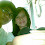## Wednesday, February 29, 2012

### How to convert string to integer in Arduino?

Today just found myself having to convert a string to an integer in an Arduino. Had a code that did character to integer but not from string. So copy from here to there ended up with this function. And posted here just for anybody to use or have a starting point.
Here is the code, use it change it or don't use it. Hope this saves you some time.
`````` int8_t satoi( char *str, int len ){
int8_t r = 0;
for(int i=0; i<len; i++){
//Check if this is a number
if ( str[i] < 0x3a && str[i] > 0x2f){
// is a ASCII number, return it
r = r * 10;
r += (str[i]-0x30);
}else{
i = len; //exit!
r = -1;
break;
}
}
return r;
}
int8_t satoi( char *str ){
int8_t r = 0;
int len = strlen(str);
for(int i=0; i<len; i++){
//Check if this is a number
if ( str[i] < 0x3a && str[i] > 0x2f){
// is a ASCII number, return it
r = r * 10;
r += (str[i]-0x30);
}else{
i = len; //exit!
r = -1;
break;
}
}
return r;
}
``````

1.nice job bro...
I nedd it, Thanks..
can i copy to my blog??
YES U can..
owhh Thank u...

2.// convert arduino String to int
int stringToInt(String s){

char char_string[s.length()+1];
s.toCharArray(char_string, s.length()+1);

return atoi(char_string);
}